# Benjamin-Bona-Mahony equation

(diff) ← Older revision | Latest revision (diff) | Newer revision → (diff)

BBM equation, regularized long wave equation

The model equation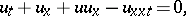(a1)

where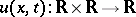and the subscripts denote partial derivatives with respect to timeand the position coordinate. The Benjamin–Bona–Mahony equation serves as an approximate model in studying the dynamics of small-amplitude surface water waves propagating unidirectionally, while suffering non-linear and dispersive effects. (a1) was introduced in [a5] as an alternative of the famous Korteweg–de Vries equation(a2)

Unlike the Korteweg–de Vries equation, the Benjamin–Bona–Mahony equation is not integrable by the inverse scattering method [a10], [a12]. As indicated by several numerical experiments, (a1) has no multi-soliton solutions. It has been proved by A.C. Bryan and A.E.G. Stuart [a8] that (a1) has no analytic two-soliton solution. The equation has three independent invariants (conservation laws):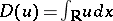;; and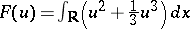. These quantities are time-independent during the time evolution of the solution. The correctness of the initial value problem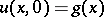(the Cauchy problem) for (a1) in Sobolev spaces,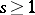(cf. also Sobolev space), was investigated in [a5].

Equation (a1) has a solitary wave solution, where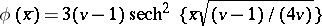(cf. also Soliton), provided that the wave velocity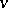satisfies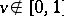. The non-linear stability of the wavewith respect to the pseudo-metric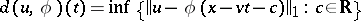was established in [a3] and [a7] by a clever modification of Lyapunov's direct method in combination with a spectral decomposition technique. Here,is the norm in the Sobolev space. This means that the form of the solitary wave is stable under small perturbations in the form of the initial wave.

## Generalizations.

The generalized Benjamin–Bona–Mahony equation is an equation of the form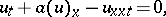(a3)

whereis a differentiable function. (a3) allows two types of solitary waves: kink-shaped and bell-shaped ones. Depending on the concrete form of the non-linearity, these solitary waves can be stable or unstable with respect to the metric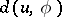. For more concrete results concerning (a3), see [a11], Chap. 4. The generalized Benjamin–Bona–Mahony equation in higher dimensions reads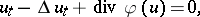(a4)

whereis the Laplace operator in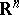and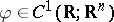. The uniqueness and global existence of a solution in Sobolev spaces to the initial boundary value problem for (a4) in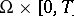, with Dirichlet (or more general) boundary conditions, was proved in [a2], [a9]. Here,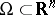is a bounded domain with smooth boundary. The Cauchy problem for (a4) is studied in [a1].

Non-local generalizations of the Benjamin–Bona–Mahony equation can be obtained after one writes (a1) in the form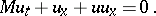Here,is a pseudo-differential operator (in fact, a Fourier multiplier operator), acting as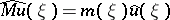, where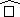denotes the Fourier transform in the space variable. For the original Benjamin–Bona–Mahony equation one has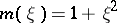. In general, one takes fora positive even function such that its negative poweris monotone decreasing on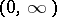and belongs to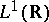. See [a4], [a5] and the references therein for more details.

The variable-coefficient Benjamin–Bona–Mahony equation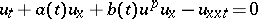describes the propagation of long weakly non-linear water waves in a channel of variable depth. This equation was studied in [a6].

How to Cite This Entry:
Benjamin-Bona-Mahony equation. Encyclopedia of Mathematics. URL: http://encyclopediaofmath.org/index.php?title=Benjamin-Bona-Mahony_equation&oldid=15681
This article was adapted from an original article by Iliya D. Iliev (originator), which appeared in Encyclopedia of Mathematics - ISBN 1402006098. See original article